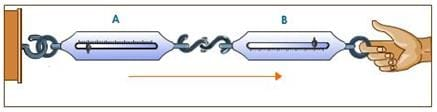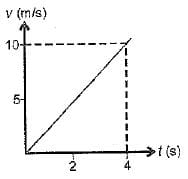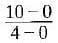# MCQ : Force And Laws Of Motion - 1

## 10 Questions MCQ Test Science & Technology for UPSC CSE | MCQ : Force And Laws Of Motion - 1

Description
Attempt MCQ : Force And Laws Of Motion - 1 | 10 questions in 10 minutes | Mock test for Class 9 preparation | Free important questions MCQ to study Science & Technology for UPSC CSE for Class 9 Exam | Download free PDF with solutions
QUESTION: 1

### Newton’s third law of motion explains the two forces namely ‘action’ and ‘reaction’ coming into action when the two bodies are in contact with each other. These two forces:Solution:

Always act on the different bodies in opposite directions.

QUESTION: 2

### The inertia of an object tends to cause the object ______.

Solution:

Inertia of an object is the tendency of the object to resist change in motion. The inertia is directly dependent on the mass of the body.
Inertia resists any change in its state of motion and is of three types:

• Inertia of rest
• Inertia of motion
• Inertia of direction
QUESTION: 3

### While catching a stone thrown by your friend you pull the hands back to______.

Solution:
• While catching a fast moving cricket ball, a fielder in the ground gradually pulls his hand backward with the moving ball, in doing so, the fielder increases the time during which the high velocity of the moving ball decrease to zero.
• This, the acceleration of the ball is decreased and therefore, the impact of catching the fast moving ball is also reduced.
QUESTION: 4

Which one of the following statements is not correct for an object moving along a straight path in an accelerated motion?

Solution:
• The question states an accelerated motion of an object along a straight line, this implies a non zero force is acting on the object, which is increasing its velocity and speed.
• However the direction of force is not given so it can’t be determined that it is moving away from the earth, Hence the correct answer is C.
QUESTION: 5

The v - t graph of a body of 5 kg moving with the help of a force is shown. Then the force involved is______.Solution:

a = slope of graph == 2.5 m/s2
F = ma = 5 x 2.5 = 12.5 N
(c) is correct.

QUESTION: 6

Force is defined as:

Solution:

"The rate of change of momentum is called force”. “ When a net force acts on a body, it produces acceleration in thr body and will be equal to rate of change of momentum”. Hence , we found that Rate of change of momentum is equal to Force applied on the object .

QUESTION: 7

When no external force acts on an object, the physical quantity that remains conserved is______.

Solution:

If no external force applied then acceleration will be zero, so velocity remain conserve.
Momentum = mass * velocity, if velocity don't Change momentum also not change.

QUESTION: 8

The acceleration of an object is______.

Solution:

Newton's second law of motion can be formally stated as follows:- The acceleration of an object as produced by a net force is directly proportional to the magnitude of the net force, in the same direction as the net force, and inversely proportional to the mass of the object.

QUESTION: 9

According to second law of Newton, force is the cause and the outcome is______.

Solution:

According to Newton’s second law of motion, also known as the Law of Force and Acceleration, a force upon an object's causes it to accelerate according to the formula:
Net force = mass x acceleration.

So, the acceleration of the object is directly proportional to the force and inversely proportional to the mass.

QUESTION: 10

Which of the following is an equation of motion of a body?

Solution:

Newton's second law, which states that the force F acting on a body is equal to the mass m of the body multiplied by the acceleration a of it's centre of mass, F= ma, is the basic equation of motion in classical mechanics.Use Code STAYHOME200 and get INR 200 additional OFF Use Coupon Code